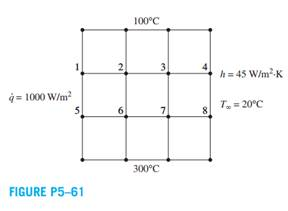### Create an Account

Home / Questions / Consider steady two-dimensional heat conduction in a square cross section 3 cm 3 cm k

# Consider steady two-dimensional heat conduction in a square cross section 3 cm 3 cm k

Consider steady two-dimensional heat conduction in a square cross section (3 cm × 3 cm, k = 20 W/m·K, α = 6.694 × 10-6 m2/s) with constant prescribed temperature of 100°C and 300°C at the top and bottom surfaces, respectively. The left surface is exposed to a constant heat flux of 1000 W/m2 while the right surface is in contact with a convective environment (h = 45 W/m2·K) at 20°C. Using a uniform mesh size of ∆x = ∆y, determine

(a) finite difference equations and

(b) the nodal temperatures using Gauss-Seidel iteration method.Jul 20 2020 View more View LessSubscribe To Get Solution